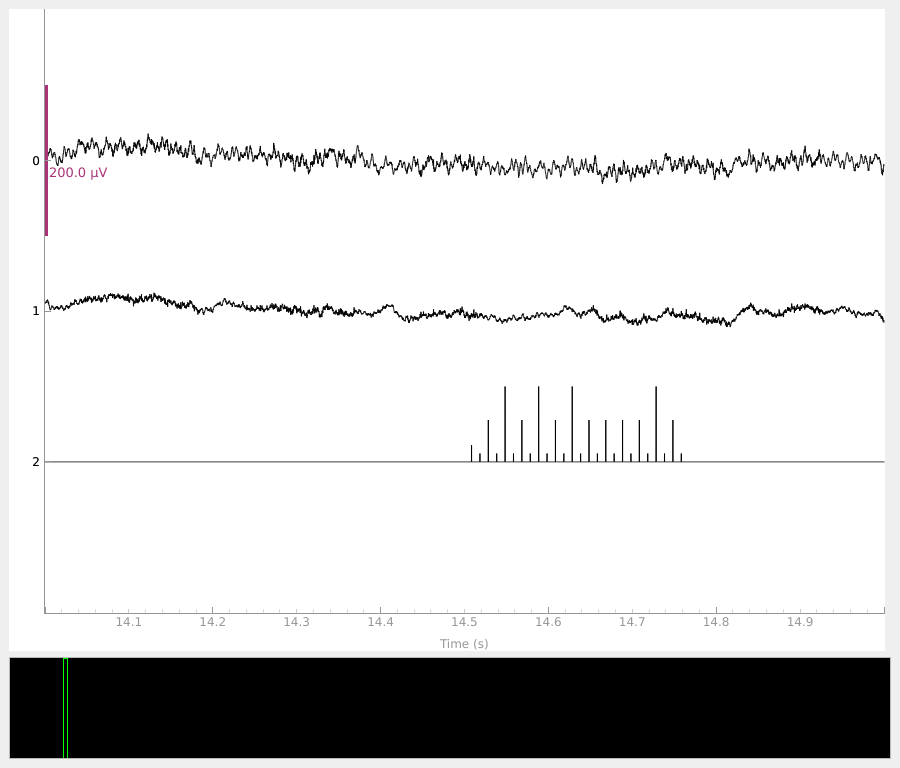Here we read some sample XDF data. Although we do not analyze it here, this recording is of a short parallel auditory response (pABR) experiment 1 and was provided by the Maddox Lab.

```# Authors: Clemens Brunner <clemens.brunner@gmail.com>
#          Eric Larson <larson.eric.d@gmail.com>
#
```
```import pyxdf

import mne
from mne.datasets import misc

fname = misc.data_path() / "xdf" / "sub-P001_ses-S004_task-Default_run-001_eeg_a2.xdf"
data = streams["time_series"].T
assert data.shape == 5  # four raw EEG plus one stim channel
data[:4:2] -= data[1:4:2]  # subtract (rereference) to get two bipolar EEG
data = data[::2]  # subselect
data[:2] *= 1e-6 / 50 / 2  # uV -> V and preamp gain
sfreq = float(streams["info"]["nominal_srate"])
info = mne.create_info(3, sfreq, ["eeg", "eeg", "stim"])
raw = mne.io.RawArray(data, info)
raw.plot(scalings=dict(eeg=100e-6), duration=1, start=14)
``````Creating RawArray with float64 data, n_channels=3, n_times=2320128
Range : 0 ... 2320127 =      0.000 ...   232.013 secs